{{announcement.body}}
{{announcement.title}}

# Dynamic Programming or 'Divide and Conquer'

DZone 's Guide to

# Dynamic Programming or 'Divide and Conquer'

### In this post, we look at the computer science behind dynamic programming, and how dynamic programming can be used in JS code.

· Web Dev Zone ·
Free Resource

Comment (0)

Save
{{ articles.views | formatCount}} Views

The comparison will be made with the example of two algorithms: binary search (how to quickly find the number in the sorted array) and Levenshtein distances (how to convert one string to another with the minimum number of operations).

This article is just my personal attempt to decompose everything into shelves and understand what dynamic programming is and how the "divide and conquer" principle participates in it.

## Problems

When I began to study algorithms, it was difficult for me to understand the basic idea of dynamic programming (hereinafter DP, from Dynamic Programming) and how it differs from the divide-and-conquer (DC) approach. When it comes to comparing these two paradigms, usually many people successfully use the Fibonacci function for illustration. And this is an excellent illustration. But it seems to me that when we use the same task to illustrate DP and DC, we lose one important detail that can help us to grasp the difference between the two approaches more quickly. And this detail is that these two techniques are best manifested in solving different types of problems.

I'm still in the process of studying DP and DC and I can not say that I completely understood these concepts. But I still hope that this article will shed additional light and help in studying such important approaches as dynamic programming and divide-and-conquer.

## The Similarity Between DP and DC

The way I see these two concepts now, I can conclude that DP is an extended version of DC.

I would not consider them as something completely different. Because both of these concepts recursively break the problem into two or more sub-problems of the same type and re-type, until these sub-problems are light enough to solve them "directly." Further, all decisions of sub-problems are combined together in order to finally answer the original, original problem.

So why then do we still have two different approaches (DP and DC) and why I called dynamic programming an extension. This is because dynamic programming can be applied to tasks that have certain characteristics and constraints. And only in this case, DP expands the DC through two techniques: memoization and tabulation.

Let's go a little deeper into the details...

### Limitations and Characteristics Necessary for Dynamic Programming

As we have just found out, there are two key characteristics that a task/problem must have in order for us to try to solve it using dynamic programming:

• The optimal sub-structure: It should be possible to compose the optimal solution to the problem from the optimal solution of its subproblems.

• Intersecting sub-problems: The problem must be broken down into sub-problems, which in turn are re-used. In other words, a recursive approach to solving a problem would imply multiple (not single) solutions of the same sub-problem, instead of generating all the new and unique sub-problems in each recursive cycle.

As soon as we can find these two characteristics of the problem we are considering, we can say that it can be solved using dynamic programming.

## Dynamic Programming, as an Extension of the "Divide and Conquer" Principle

DP extends the DC with the help of two techniques (memoization and tabulation), the purpose of which is to preserve the solutions of sub-problems for their further reuse. Thus, the solutions to sub-problems are cached, which leads to a significant improvement in the performance of the algorithm. For example, the time complexity of the "naive" recursive implementation of the Fibonacci function is O (2n). While a solution based on dynamic programming is executed just in time O (n).

Memoization (filling the cache from top to bottom) is a caching technique that re-uses previously calculated sub-tasks. The Fibonacci function using the technique of memoization would look like this:

``````memFib(n) {
if (mem[n] is undefined)
if (n < 2) result = n
else result = memFib(n-2) + memFib(n-1)
mem[n] = result
return mem[n]
}``````

Tabulation (filling the cache from the bottom-up) is a similar technique, but which is primarily focused on filling the cache, rather than on finding a solution to the sub-problem. Calculating the values that need to be placed in the cache is easiest, in this case, to perform iteratively, rather than recursively. The Fibonacci function using tabulation would look like this:

``````tabFib(n) {
mem = 0
mem = 1
for i = 2...n
mem[i] = mem[i-2] + mem[i-1]
return mem[n]
}``````

More details about the comparison of memoization and tabulation can be found here.

The main idea that should be caught in these examples is that since our DC problem has overlapping sub-problems, we can use caching solutions to sub-problems using one of two caching techniques: memoization and tabulation.

## The Difference Between DP and DC

We got acquainted with the limitations and prerequisites of using dynamic programming, as well as caching techniques used in the DP approach. Let's try to summarize and depict the above thoughts in the following illustration: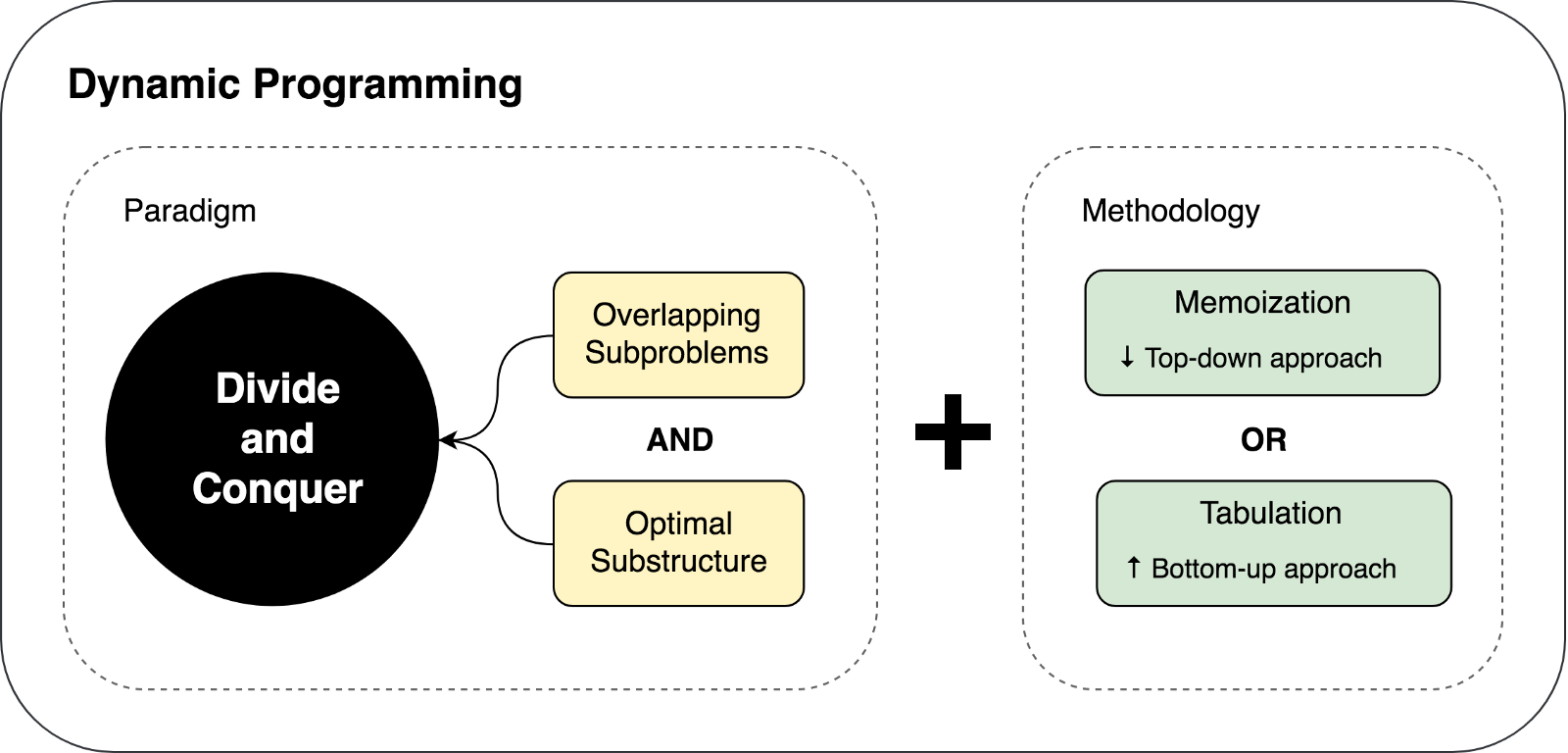Let's try to solve a couple of tasks using DP and DC to demonstrate both of these approaches in action.

An example of the "divide and conquer" principle: binary search.

The binary search algorithm is a search algorithm that finds the position of the requested element in the sorted array. In binary search, we compare the value of the required variable with the value of the element in the middle of the array. If they are not equal, then half the array in which the element you are looking for cannot be excluded from the further search. The search continues in the half of the array in which the desired variable can be found until it is found. If the next half of the array does not contain elements, the search is considered complete and we conclude that the array does not contain the desired value.

## Example

In the illustration below, an example of a binary search for the number 4 in an array.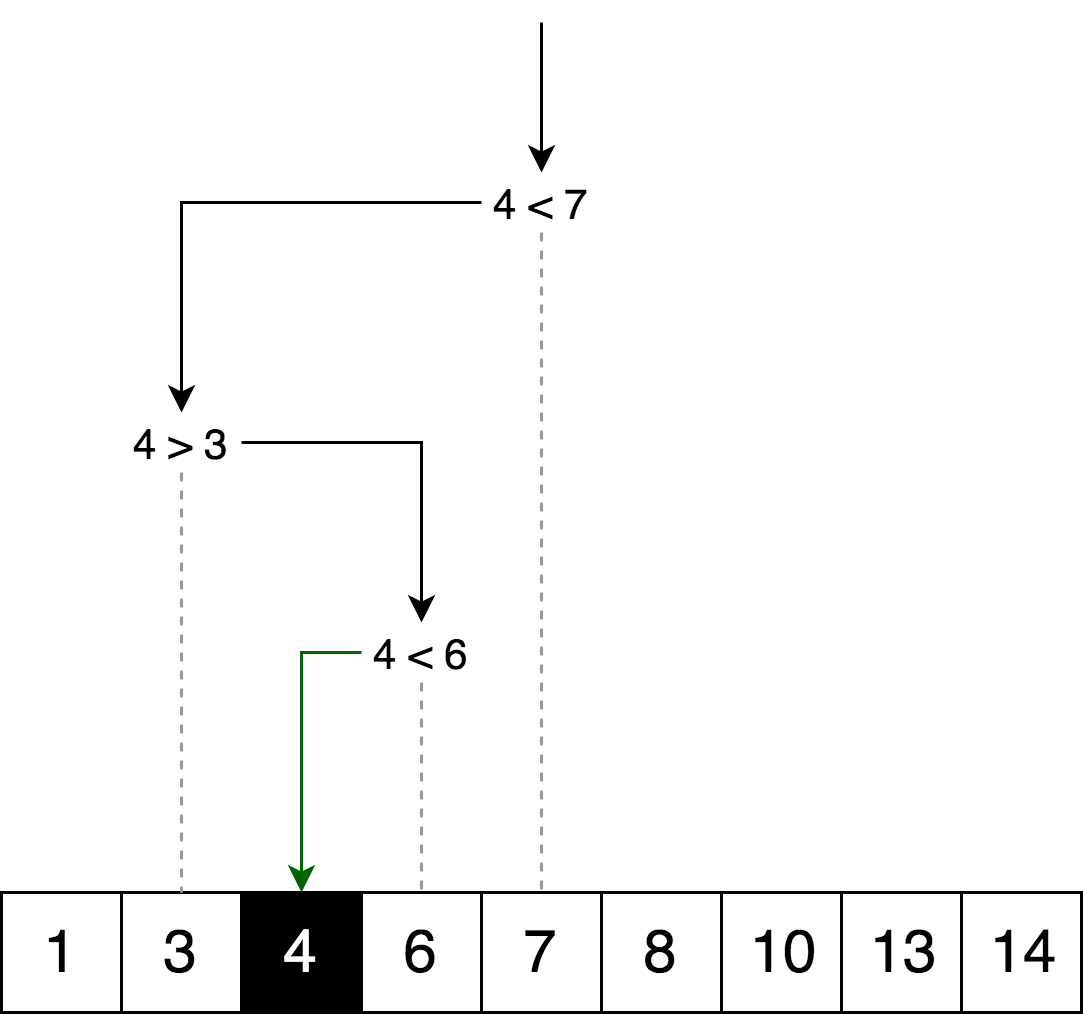Let's display the same search logic but in the form of a decision tree.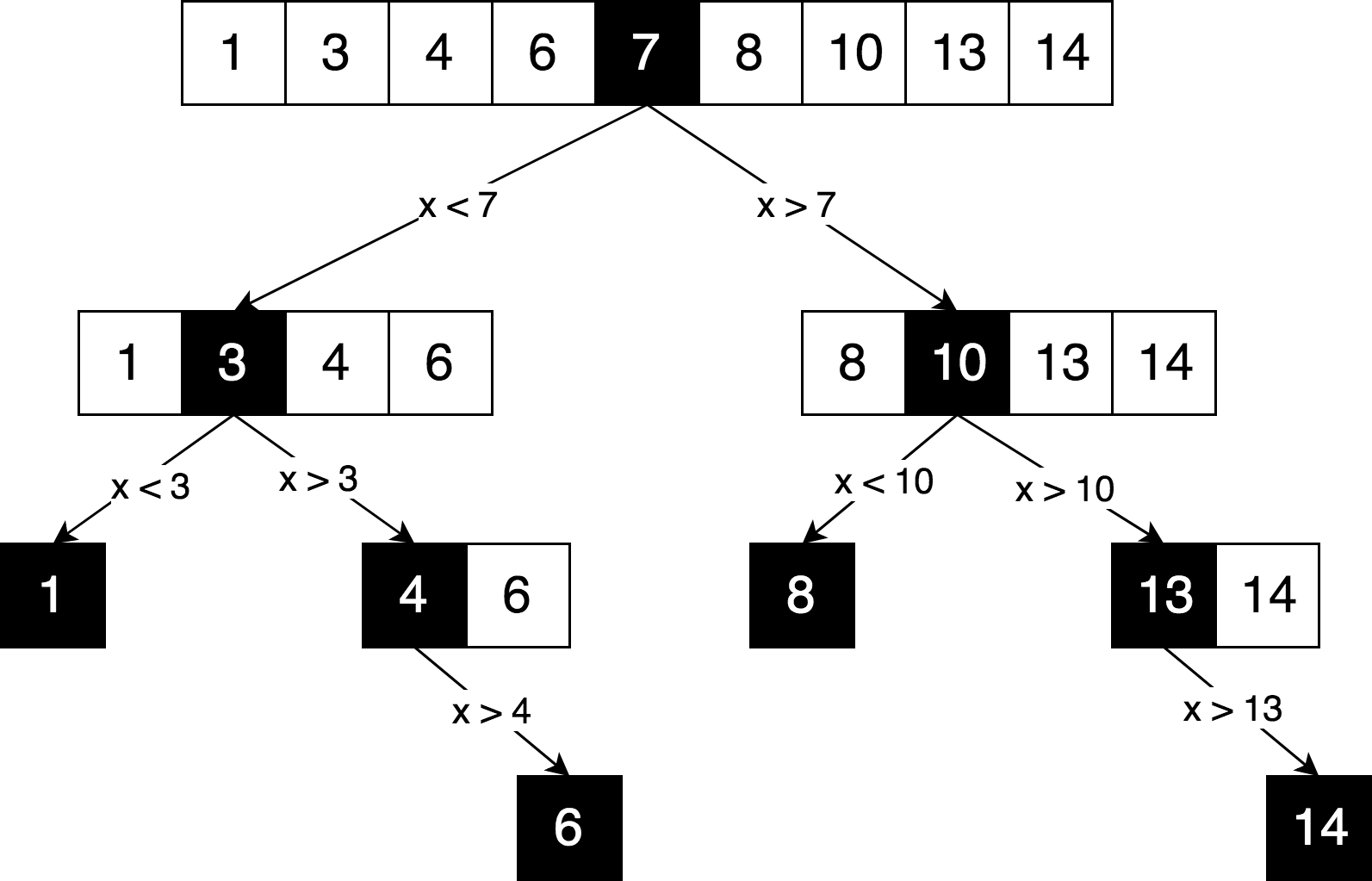You can see in this scheme a clear principle of "divide and conquer", used to solve this problem. We iteratively break our original array into sub-arrays and try to find the desired element already in them.

Can we solve this problem using dynamic programming? No. For the reason that this problem does not contain intersecting sub-problems. Every time we split an array into parts, both parts are completely independent and non-intersecting. And according to the assumptions and limitations of dynamic programming, which we discussed above, sub-problems must somehow intersect, they must be repetitive.

Usually, whenever the decision tree looks just like a tree (rather than as a graph), it most likely means the absence of overlapping sub-problems,

## Implementation of the Algorithm

Here you can find the full source code of the binary search algorithm with tests and explanations.

``````function binarySearch(sortedArray, seekElement) {
let startIndex = 0;
let endIndex = sortedArray.length - 1;
while (startIndex <= endIndex) {
const middleIndex = startIndex + Math.floor((endIndex - startIndex) / 2);
// If we've found the element just return its position.
if (sortedArray[middleIndex] === seekElement)) {
return middleIndex;
}
// Decide which half to choose: left or right one.
if (sortedArray[middleIndex] < seekElement)) {
// Go to the right half of the array.
startIndex = middleIndex + 1;
} else {
// Go to the left half of the array.
endIndex = middleIndex - 1;
}
}
return -1;
}``````

## Example of Dynamic programming: Editing Distance

Usually, when it comes to explaining dynamic programming, the Fibonacci function is used as an example. But, in our case, let's take a slightly more complicated example. The more examples, the easier it is to understand the concept.

Editing distance (or Levenshtein distance) between two lines is the minimum number of insertion operations of one character, the removal of one character and the replacement of one character for another, necessary for converting one line to another.

For example, the editing distance between the words "kitten" and "sitting" is 3, since you need to perform three editing operations (two replacements and one insertion) to convert one string to another. And it is impossible to find a faster version of the conversion with fewer operations:

kitten → sitten (replacing "k" with "s")
sitten → sittin (replacing "e" with "i")
sittin → sitting (insert "g" completely).

## Application of the Algorithm

The algorithm has a wide range of applications, for example, for spell checking, optical recognition correction systems, inaccurate string search, etc.

### The Mathematical Definition of the Problem

Mathematically, the distance between Levenstein between two rows a, b (with the lengths | a | and | b |, respectively) defines function lev (| a |, | b |), where: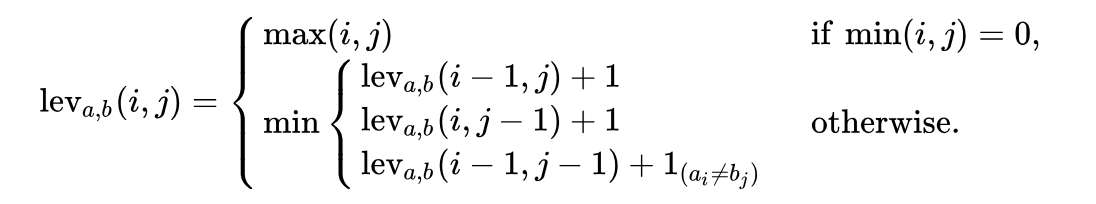Note that the first line in the min function corresponds to the delete operation, the second line corresponds to the insertion operation and the third line corresponds to the replacement operation (in case the letters are not equal).

### Explanation

Let's try to understand what this formula tells us. Let's take the simple example of finding the minimum editing distance between the lines ME and MY. Intuitively, you already know that the minimum edit distance is one (1) replacement operation (replace "E" with "Y"). But let's formalize our decision and turn it into an algorithmic form, in order to be able to solve more complex versions of this problem, such as the transformation of the word Saturday into Sunday.

In order to apply the formula to ME ... MY transformation, we first need to know the minimum editing distance between ME → M, M → MY and M → M. Next, we must choose from three distances the minimum and add to it one operation (+1) of the transformation E → Y.

So, we can already see the recursive nature of this solution: the minimum editing distances ME → MY is calculated on the basis of the three previous possible transformations. In this way, we can already say that this is the "divide and conquer" algorithm.

To further explain the algorithm, let's put our two lines in the matrix: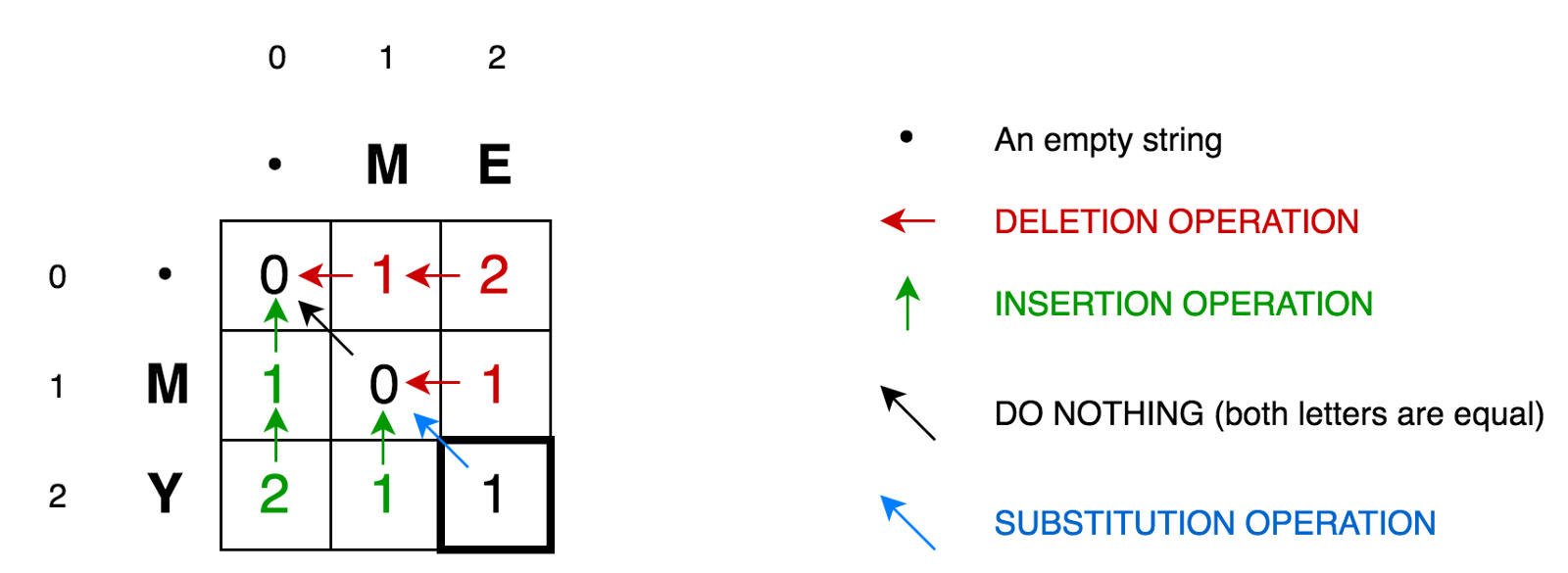The cell (0,1) contains the red number 1. This means that we need to perform 1 operation in order to convert M to an empty string — to delete M. Therefore, we have designated this number in red.

The cell (0.2) contains the red number 2. This means that we need to perform 2 operations in order to transform the ME string into an empty string — delete E, delete M.

The cell (1,0) contains the green number 1. This means that we need 1 operation to transform the empty string into M — insert M. We marked the insertion operation in green.

Cell (2,0) contains a green number of 2. This means that we need to perform 2 operations in order to convert an empty string into a string MY - insert Y, insert M.

The cell (1,1) contains the number 0. This means that we do not need to do any operations, in order to convert the string M into M.

The cell (1,2) contains the red number 1. This means that we need to perform 1 operation to transform the string ME into M  — delete E

And so on…

It does not look complicated for small matrices, such as ours (only 3x3). But how can we calculate the values of all the cells for large matrices (for example, for the matrix 9x7 with the transformation Saturday → Sunday)?

The good news is that, according to the formula, everything we need to calculate the value of any cell with coordinates (i, j) is just the values of 3 neighboring cells (i-1, j), (i-1 , j-1), and (i, j-1). All we need to do is find the minimum value of three adjacent cells and add one (+1) to this value in case we have different letters in the i-th row and j-th column.

So, again, you can clearly see the recursive nature of this task.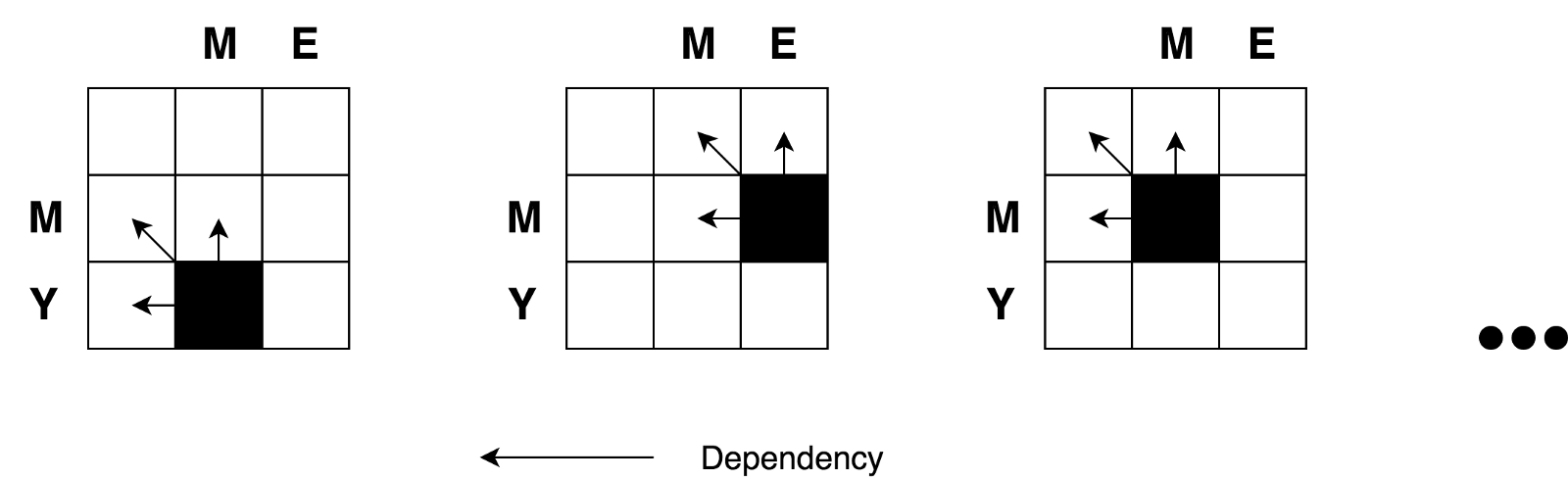We also saw that we are dealing with a task like "divide and conquer." But, can we apply dynamic programming to solve this problem? Does this task satisfy the above-mentioned conditions of "overlapping problems" and "optimal sub-structures"? Yes. Let's build a decision tree.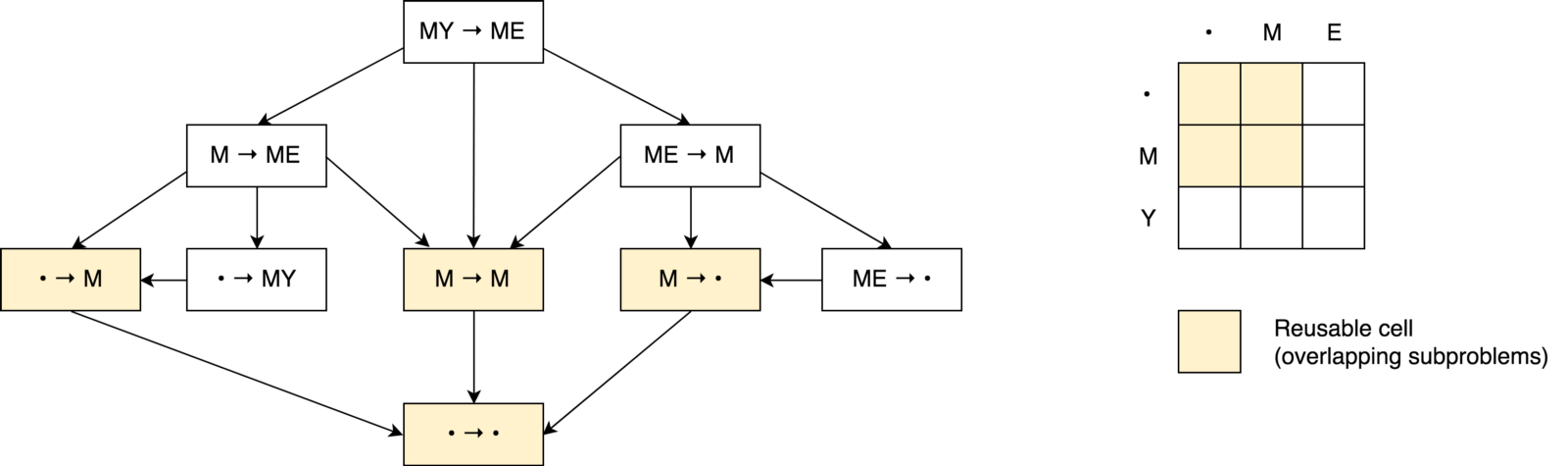First, you may notice that our decision tree looks more like a solution graph than a tree. You can also notice several overlapping sub-tasks. It is also clear that it is impossible to reduce the number of operations and make it smaller than the number of operations from those three neighboring cells (sub-problems).

You can also notice that the value in each cell is calculated based on the previous values. Thus, in this case, the tabulation technique is used (filling the cache in the direction from the bottom to the top). You will see this in the code example below. Applying all these principles, we can solve more complex problems, for example, the transformation task Saturday → Sunday: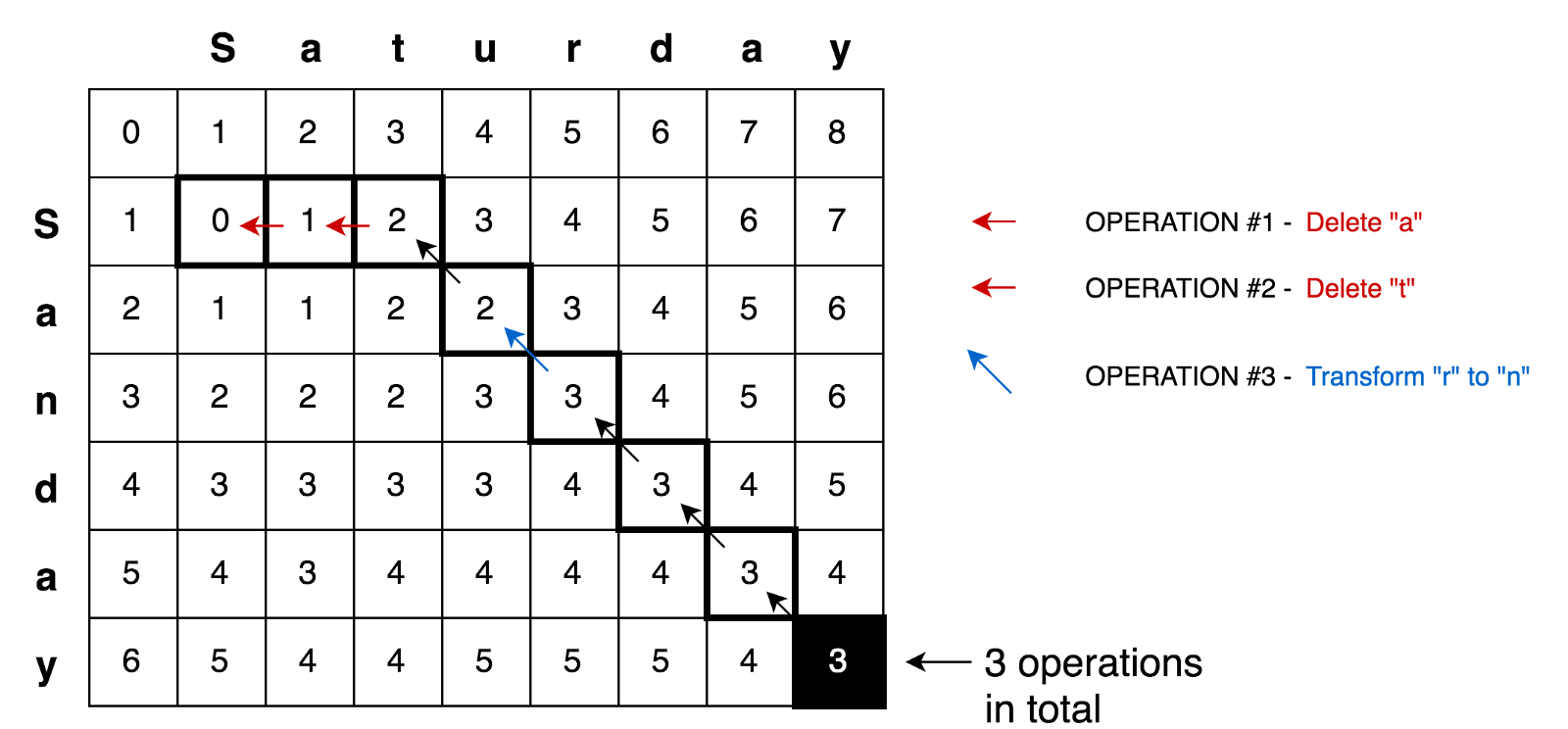## Sample Code

Here you can find the complete solution for finding the minimum editing distance with tests and explanations:

``````function levenshteinDistance(a, b) {
const distanceMatrix = Array(b.length + 1)
.fill(null)
.map(
() => Array(a.length + 1).fill(null)
);
for (let i = 0; i <= a.length; i += 1) {
distanceMatrix[i] = i;
}
for (let j = 0; j <= b.length; j += 1) {
distanceMatrix[j] = j;
}
for (let j = 1; j <= b.length; j += 1) {
for (let i = 1; i <= a.length; i += 1) {
const indicator = a[i - 1] === b[j - 1] ? 0 : 1;

distanceMatrix[j][i] = Math.min(
distanceMatrix[j][i - 1] + 1, // deletion
distanceMatrix[j - 1][i] + 1, // insertion
distanceMatrix[j - 1][i - 1] + indicator, // substitution
);
}
}
return distanceMatrix[b.length][a.length];
}``````

## Conclusions

In this article, we compared two algorithmic approaches ("dynamic programming" and "divide and conquer") to solving problems. We found that dynamic programming uses the "divide and conquer" principle and can be applied to solving problems if the problem contains intersecting sub-problems and an optimal sub-structure (as in the case of the Levenshtein distance). Dynamic programming then uses memo and tabulation techniques to store sub-solutions for their re-use.

I hope this article rather clarified, but not complicated the situation for those of you who have tried to deal with such important concepts as dynamic programming and "divide and conquer."

You can find more algorithmic examples using dynamic programming, with tests and explanations in the JavaScript Algorithms and Data Structures repository.

Successful coding!

Topics:
common lisp, dynamic programming, javascript, programming practices, web dev

Comment (0)

Save
{{ articles.views | formatCount}} Views

Opinions expressed by DZone contributors are their own.